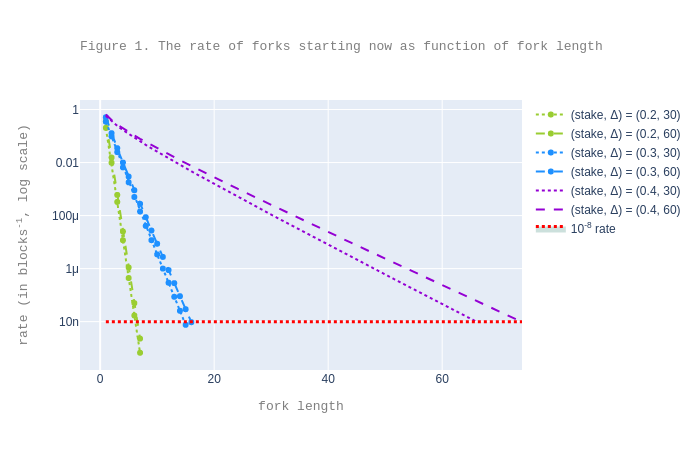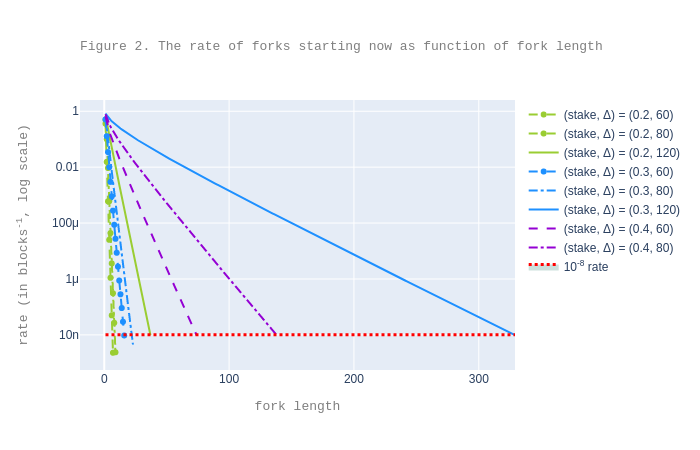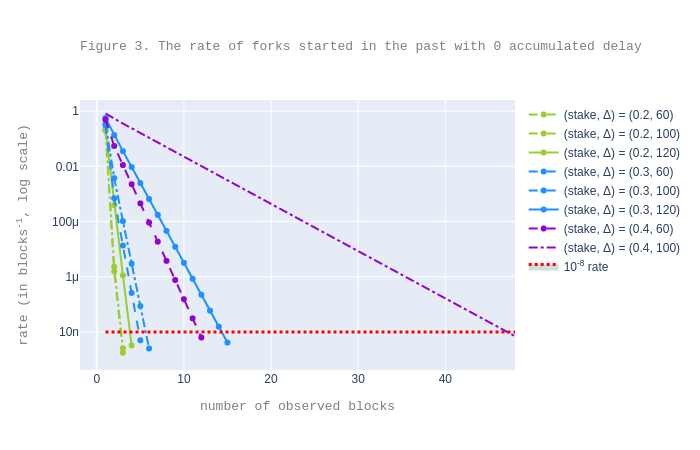1. Research & development
2. > Blog >
3. Emmy+ in the partial synchrony model

# Emmy+ in the partial synchrony model

in-depth
09 October 2020
share:

We’d like to expand on our previous blog post analysing Emmy+.

Note: Thanks to input from Bruno Blanchet (Inria). The interested reader can experiment with our code used in the analysis. As in the previous analysis, we do not present any security proofs.

## To recap

Emmy+ is the new Tezos consensus algorithm, adopted in October 2019. Emmy+ improved on the original Emmy (see our post on Emmy+: an improved consensus algorithm).1

In our previous analysis of Emmy+ we assumed a perfect network that delivers blocks and operations instantly to all participants. That is, using the terminology below: we assumed a synchronous model with zero message delivery time. This simplifying assumption was shared with the original analyses of both Bitcoin and Ouroboros.

Now we will refine our previous assumption to something more realistic: the partial synchrony network model.

In brief: $$\newcommand\mdelta{{{\Delta}}} \newcommand{\mtype}{{\mathtt{#1}}} \newcommand\ie{\mathit{ie}} \newcommand\dpp{\mathit{dp}} \newcommand\de{\mathit{de}} \newcommand\edelay{\mathit{emmy\_delay}} \newcommand\edelaydelta{\edelay_{\Delta}}$$

1. In a synchronous model, message delivery delay is bounded by a known bound $$\mdelta$$. A message sent at time $$t$$ will be delivered by time $$t+\mdelta$$.
2. In an asynchronous network model, message delivery time is unbounded (but each message must eventually be delivered).
3. In a partially synchronous model, a global bound $$\mdelta$$ exists, but its value is unknown.2

Note: There are two equivalent formulations of the partially synchronous model: unknown latency (as above), and the global stabilization time (GST) flavor. We will briefly discuss GST towards the end of the post.

## Minimal block delay in partial synchrony

So this blog post profiles Emmy+ in a partially synchronous network.

Emmy+ defines a minimal block delay function as a function of the current block’s priority $$p$$, and the number of endorsements $$e$$ included in the current block:

$$\edelay(p, e) = 60 + \dpp \cdot p + \de\cdot \max(0, \ie - e) .$$

Above

• $$\dpp$$ stands for “delay per priority” (that is, per missed baking slot),
• $$\de$$ stands for “delay per (missed) endorsement (slot)”,
• $$\ie$$ stands for number of initial endorsements, and
• the constant 60 represents the minimal time between the blocks.

The previous analysis of Emmy+ effectively set $$\mdelta$$ to 0, so the same function could be used for the analysis. Now to account for partial synchrony, we elaborate $$\edelay$$ to capture message delays. We consider a worst case scenario, in which the network maximally favors the attacker, thus:

• the honest baker’s messages get delayed by the maximum possible $$\mdelta$$ while
• the attacker’s messages arrive instantly.

In symbols, the minimal block delay function $$\edelaydelta(p, e)$$ becomes

• $$\edelaydelta(p, e) = \min(\max(2\mdelta, \edelay(p, e)), \max(\mdelta, \edelay(p, 0)))$$ for blocks baked by an honest baker, and remains as
• $$\edelaydelta(p, e) = \edelay(p, e)$$ for blocks baked by the attacker.

The new delay function can be understood as follows:

• once a block is baked, it takes $$\mdelta$$ time units for the other honest bakers to receive it and
• it takes another $$\mdelta$$ time units to gather the endorsements for the new block to be baked, consequently
• the earliest “baking time” is
$$\max(2\mdelta, \edelay(p, e)).$$
• If the baker prefers to bake its block without endorsements, then the earliest “baking time” is
$$\max(\mdelta, \edelay(p, 0)) .$$

Observation 1: If $$\mdelta\leq 30s$$ then

$$\edelaydelta(p, e) = \edelay(p, e) .$$

So, the previous analysis also holds in a synchronous network model, provided $$\mdelta\leq 30s$$.

## Fork probabilities

As in our previous analysis, we consider a Byzantine attacker — so the attacker is cost-insensitive and could double sign3. The attacker wants to undo a transaction by

1. baking an alternative chain which does not include the transaction, and
2. revealing this chain at a later point, thus creating a “fork”.

The attacker’s chain must become faster than the “honest” chain to be adopted as the new chain. So basically, we must compute the probability that the timestamp of the head of the dishonest chain is less than the timestamp of the head of the honest chain. This difference can be expressed in terms of differences between minimal block delays, and this is where $$\mdelta$$ enters the analysis. See here for details.

In what follows, we analyze the impact of $$\mdelta$$ on fork probabilities. We recall that the previous analysis considered two main scenarios

• forks starting now and
• forks starting in the past,

which we will reconsider below.

In both cases, we ask when a transaction can be considered “final”, that is:

How many confirmations (blocks on top of the block containing the relevant transaction) must we observe to be reasonably sure the transaction will remain in the chain?

Here, reasonably sure means “with probability smaller than some reasonable threshold”, which we quantify as $$\small 10^{-8}$$ (which puts our expectation of being wrong about a block being final at roughly once every two centuries). We call confirmation number the expected number of confirmations for a block to be considered final.

### Forks starting now

Anybody with a small child will know that the contents of their fork may be recorded on the tablecloth, and there may only be a certain time period after which this becomes indelible.

In a similar vein we can ask of Tezos:

Given that my transaction belongs to the current blockchain head, how many confirmations do I need to see to be reasonably sure that my transaction is final?

We addressed this in our previous analysis: we provided 7, 16, 67 as confirmation numbers for .2, .3, respectively .4 attacker stake fraction and $$\mdelta= 0s$$. By Observation 1, the same holds also for $$\mdelta \leq 30s$$.

We redo our computations with the new delay function $$\edelaydelta$$ for $$\mdelta = 60s$$. We see that the confirmation numbers only increase more visibly for .4 attacker stake.However, for larger $$\mdelta$$, the data suggests that we can no longer provide a decent criterion for high attacker stake fractions. For instance, for $$\mdelta = 120s$$, already for an attacker stake fraction of 0.3, the confirmation number exceeds 300.### Forks started in the past

Now suppose we were absent from the room and returned to find our child surrounded by spilled food. After which length of absence do we know there is no hope of cleaning up?

Likewise we can ask of Tezos:

I have seen $$n$$ confirmations since my transaction was included in a block, $$d$$ time ago; is my transaction final?

We addressed this in our previous analysis: we considered the case where the accumulated delay is $$d-60 \cdot n$$, or in words: the delay of the current head with respect to the earliest time the current head could ideally have been baked, counting from the block where the transaction was included.

A more relevant case is that of a healthy network, that is, one with 0 accumulated delay (which is the usual case on the mainnet) and with $$\mdelta\leq 30s$$. Notably, in this case, the values for $$n$$ are 3, 5, and 12 for .2, .3, respectively .4 attacker stake fraction. These numbers are obtained with the same implementation as the one used in previous analysis, even though they do not appear as such there.

Remark: the fact that, at the current moment, the chances that there exists already a (covert) feasible fork (started at least $$n$$ healthy blocks ago, with $$n$$ as above) are tiny, does not mean that $$n$$ is a suitable confirmation number, because if network delays become large henceforth, then the attacker could (in theory) take advantage of this to overtake the honest chain. Put another way: if network delays are possible, then we cannot consider a block $$n$$ levels deep to be final even if $$n$$ is large and everything has gone well so far.

We now set about formalizing this remark. We first consider a variant as follows:

Assume the attacker started its chain $$n$$ blocks ago. What is the least $$n$$ such that the probability that the attacker has a faster chain if revealed now, is smaller than our security threshold $$\small10^{-8}$$?

This variant differs from the question implicit in the Remark above in that the variant disregards the future. This means that, since we assume 0 accumulated delay, the computation of the probability of forks only depends on the attacker’s stake, and not on $$\mdelta$$, because we assume that the network “works” in its favor. We obtain the following values for $$n$$, given the following stake fractions $$f$$ ($$f$$ varied in increments of $$0.005$$):

• $$n=2$$ for $$0.1 \leq f \leq 0.295$$,
• $$n=3$$ for $$0.3 \leq f \leq 0.435$$,
• $$n=4$$ for $$0.44 \leq f \leq 0.495$$.

These are small numbers, and we can show they remain small even for higher security thresholds. However, as the plot below suggests, as soon as we consider the probability of forks of any length (therefore also considering the future), the expected confirmation numbers grow rapidly with $$\mdelta$$.Finally, the following plot takes a different perspective, showing how fork probabilities grow with $$\mdelta$$, for a fixed number $$n$$ of seen blocks and when the chain was healthy during these last $$n$$ blocks. We take the same values of for $$n$$, namely 3, 5, and 12 for .2, .3, respectively .4 attacker, as obtained at the beginning of the section.We can see from the plot that fork probabilities grow rapidly with $$\mdelta$$ to a point where an attacker can build a fork at each level.

### Partial synchrony in the GST flavor

In the GST flavor of the partially synchronous model, there is first a period when the asynchrony assumption holds, followed by a period when the synchrony assumption holds. The end of the asynchrony period is called the global stabilization time (GST), which is assumed to be unknown. However, the upper bound $$\mdelta$$ on the message delay in the synchrony period is known a priori, and therefore can be used by the consensus algorithm.

All the above analysis is valid in the case when forks start after GST. However, when forks start before GST, we have no guarantees at all regarding finality: the blocks and operations of the honest parties may be delayed arbitrarily, and when this happens the attacker’s chain can take over the honest chain. Indeed, suppose that there are already $$n$$ confirmations, but that the next block is delayed for longer than the time needed by the adversary to build a fork of length $$n+1$$: the attacker’s chain will thus become the main chain.

Note that we can see the case when are before GST as a particular one of the UL flavor where $$\mdelta$$ tends towards infinity.

## Conclusions

Nakamoto-style consensus algorithms such as those implemented by the Bitcoin, Ouroboros, and Snow White protocols rely on synchrony and the knowledge of $$\mdelta$$ (which is implicit in the algorithm design) in order to bound the expected number of confirmations needed for a transaction to be considered as “final” — above, we called this a confirmation number.

This post provides empirical evidence that the same observation holds for Emmy+: we need synchrony to guarantee low confirmation numbers and fork probabilities.

The plots above contain curves for both large and small $$\mdelta$$, and illustrate that:

• for reasonable network delays, when $$\mdelta \leq 60s$$, the confirmation number increases only slightly, and mainly for higher attacker stake fractions, with respect to the case when $$\mdelta \leq 30s$$ (see Figures 1, 2, 3, and 4).
• for higher network delays, when $$\mdelta > 60s$$, the expected number of confirmations degrades quickly, even for smaller attacker stake fractions (see Figures 2, 3, and 4).

Specifically, the data suggests that to get decent expected confirmation numbers, it would be sufficient that the upper bound on message delays be less than the minimal time between blocks. In practice, this is extremely realistic: the least time between consecutive blocks is 60s, and this is a very generous practical upper bound on message delays.4 However, if the minimal time between blocks does not represent an upper bound on message delays, then the risk of forks increases significantly (see Figure D).

### Next steps

We recall two advantages of the classical BFT-like algorithms:

• They have deterministic finality: the finality of a transaction is ensured with probability 1 instead of being ensured with high probability.
• They provide the finality guarantee even when the network is asynchronous.

The price to pay for this is that we must make a stronger assumption that the attacker holds at most a third of the total stake. This trade-off may be worthwhile and we are experimenting accordingly with versions of the Tendermint algorithm, including with our in-house adaptation: Tenderbake. That’s a topic for another blog post, so stay tuned!

1. The post is from June 2019. The changes went into the Babylon proposal, which was injected in July. The proposal was accepted in October 2019.

2. In practice the difference between synchrony and partial synchrony is this: In a synchronous network $$\mdelta$$ is known so a receiver knows that if a message was not received by $$t+\mdelta$$, then it was never sent. In partial synchrony, the bound $$\mdelta$$ exists but the receiver does not know it, so the receiver cannot time out in the same way. What is usually done is to make successively increasing guesses: in this round of the algorithm we wait 10sec, in the next round 20sec, etc. Eventually there will be a round when we wait longer than $$\mdelta$$. Note that $$\mdelta$$ need not be a secret; the issue is just whether the algorithm may depend on it having a fixed, hardwired value.

3. Double signing” is a consequence of “double baking” or “double endorsing”, both of which are forbidden by the algorithm. A baker double bakes when she produces two different blocks at the same level. A baker double endorses when she endorses two different blocks at the same level. We recall that both baking and endorsing require the baker to sign the relevant block.

4. The 60s upper bound is extremely realistic on an average day, but perhaps one day a year it may not be. That’s one of the problems with assuming synchrony.